• matlab非线性优化函数，解决矩形材料上截取若干小圆形铁片问题，solve_simple.m是主程序，有一定实际意义。
• 结合工作经验和MATLAB帮助文档，对MATLAB非线性优化函数fmincon做了详细整理
• 优化成功或失败 一、求解失败 1、在到达迭代次数阈值或目标函数估值次数阈值时，求解器没有最小化目标到要求的精度，此时求解器停止。接下来，可以尝试以下方法： （1）设置‘Display’为‘iter’，查看每步的...

本文由olivewy编写，原地址：http://www.cnblogs.com/olivewy/p/5148428.html

优化成功或失败
一、求解失败
1、在到达迭代次数阈值或目标函数估值次数阈值时，求解器没有最小化目标到要求的精度，此时求解器停止。接下来，可以尝试以下方法：
（1）设置‘Display’为‘iter’，查看每步的迭代信息，这些信息包括：目标函数（Fval or f(x) or Resnorm）是否是下降的；检查约束越界（Max constraint）是否是递减趋向于0；查看一阶优化是否是递减趋向于0；查看置信域半径(Trust-region radius)是否下降趋向于一个小的值。若其中至少一种情况为是，就表示结果是不断改善的。如果结果是不断改善的，可以采取下边的措施：设置MaxIter、MaxFunEvals比默认值大的值，默认值可以在优化工具箱或求解器的函数参考页的优化表中查看；从最后计算出的点开始重新求解。如果结果没有改善，尝试以下其他的方法。
（2）放松精度
如果TolX或TolFun太小，当求解器达到一个最小值时可能也不会识别到，这就会导致无限次徒劳的迭代。DiffMaxChange和DiffMinChange选项能影响求解器的改善，它们控制求导估计中有限差分的步长。
（3）从不同的初始点重新开始求解
（4）检查目标函数和约束函数的定义
举个例子，可以检查目标函数和非线性约束函数在某些特定点处返回正确的值。不可行的点不一定导致函数的错误。
（5）对问题进行中心化和标准化
当每个坐标轴对目标函数和约束函数有相同的影响时，求解器更能可靠的运行，对每个坐标轴方向乘以合适的量使得每个坐标轴的影响相同，在特定的坐标轴上加上合适的值使得它们长度一致。
（6）提供解析的梯度和雅可比矩阵
如果用户不提供解析的梯度或雅可比矩阵，求解器会用有限差分来估计这些值，因此提供这些导数可以减少运算时间，提高计算准确度。
对于约束问题，提供梯度还有另一个好处----求解器到达一个点x时能满足该点是可行的，但有限差分在x点周围可能会导致不可行的点，在这种情况下，求解器可能会失败或突然中断。
（7）提供海塞矩阵
当提供海塞矩阵时，求解器能运行的更可靠，而且运行的次数比较少。
2、无可行点
在TolCon约束精度内，求解器不能找到一个满足所有约束条件的点，此时，可以尝试以下方法：
（1）检查线性约束
通过求解一个线性规划问题来找到一个满足界约束和线性约束的点。
i)定义一个目标函数是常值0的线性规划问题
f = zeros(size(x0));   % assumes x0 is the initial point
ii)求解这个线性规划问题看是否有一个可行点
xnew = linprog(f,A,b,Aeq,beq,lb,ub);
iii)如果有可行点xnew，用xnew作为初始点去求解原始问题
iv)如果没有可行点，那说明原始模型建的不好，检查界约束和线性约束。
（2）检查非线性约束
在保证界约束和线性约束是可行的之后，检查非线性约束：
i)设置目标函数为0，然后求解优化问题，如果能找到一个可行点xnew，令x0=xnew返回到原始问题中去
ii)如果用0目标函数不能找到一个可行点，尝试几个不同的初始点重新求解，如果找到了一个可行点xnew，令x0=xnew并返回到原始问题中去，如果仍没找到可行点，试着用下列方法放松约束条件。
a.改变非线性约束函数c为c-Δ，Δ是一个正数，这会使得非线性约束更容易满足。
b.尝试用原始的目标函数或0目标函数对新的约束函数寻找一个可行点。如果找到一个可行点，那么减少Δ，并在之前找到的点处开始对新的约束函数重新找一个可行点；如果没有找到一个可行点，试着增大Δ并重新找。
如果一直没有找到可行点，那么原始问题可能确实是不可行的，重新检查约束函数的定义。
3、问题是无界的
求解器到达一个目标函数小于目标阈值界的点，那么
（1）原问题可能确实无界，即存在一系列满足问题约束的点xi，使得lim f(xi) = –∞。
（2）检查原问题建模正确，求解器是最小化目标函数，如果想得到最大化，将目标函数乘以-1.
（3）试着标准化或中心化原问题。
（4）放松目标函数界精度，用optimset减少ObjectiveLimit设定的精度值。
二、求解可能成功
1、最后的点等于初始点
初始点可能是局部极小点，因为它的一阶导数接近0，如果并不确定初始点确实是一个局部极小点，尝试下边的步骤：
（1）从不同的点开始重新求解
（2）检查目标函数和约束函数定义正确。
（3）改变精度，如TolFun,TolCon,TolX
（4）标准化原问题，使得每个坐标轴有相同的影响。
（5）提供解析的梯度和海塞矩阵。
2、可能的局部最小值（Local Minimum Possible，not ' Local Minimum Found'）
求解器可能达到一个局部最小值，但并不确定，因为一阶导数不小于TolFun，为了检查得到的答案是否是可靠的，考虑下边的建议。
（1）非平滑函数
如果试着最小化一个非平滑的函数，或者有非平滑的约束，那么‘"Local Minimum Possible’是最好的返回标志，因为一阶导数条件并不适用于非平滑的点。试着检查周围的点来确定结果是否真的可靠。
（2）在最后得到的点处开始重新优化
在最后得到的点处开始重新优化会得到一个在一阶导数估量上更好的点，更好的一阶导数估量能让人相信结果是可靠的。
3、尝试一个不同的算法
4、改变精度
5、重新标准化原问题
6、检查邻近点
7、改变有限差分的计算方法
中心有限差分用的时间更多，但更准确，可以设置'FinDiffType' 为'central'。
8、提供解析的梯度或雅可比矩阵
9、提供海塞矩阵
三、求解成功
当求解器返回成功信息时，也可能结果是错误的，下边有几种方法可以验证结果。
1、改变初始点
初始点对求解结果有很大影响，如果从不同的初始点得到了相同或较差的结果，说明原来求得的界是正确的。
2、检查邻近点
计算f(xfinal±Δ)的值，与f(xfinal)值做比较。
3、检查目标函数与约束函数
4、局部最优与全局最优
（1）为什么求解器找不到最小的最小值
通常，求解器返回的是一个局部最小值，该值也可能是一个全局最小值，但并不保证是。这部分讨论求解器得出这种结果的原因，并给出寻找全局最小值的建议。
一般求解器是在初始点的basin of attraction找到的最优值，但也有些例外：
i)线性规划和正定二次规划是凸的，可行域也是凸的，所以只有一个basin of attraction。在设定某些条件下，linprog会忽略用户提供的初始点，quadprog也不需要。
ii）全局优化工具箱，如simulannealbnd，试着搜索不止一个basin of attraction。
（2）寻找一个更小的最小值
如果需要一个全局最小值，必须要在全局最小值的basin of attraction内找一个初始点。
设置初始点的建议：
i)用初始点的一个规则网格
ii）如果原始问题在所有的坐标轴上是有界的，那么从均匀分布得到一个随机初始点，如果有些部分是无界的，那么用正态、指数或其他随机分布得到初始点。对全局最优值的位置知道的越少，就要选择越分散的随机分布。例如，正态分布大部分样本在均值的三个标准偏差范围内，但柯西分布（密度函数1/(π(1 + x2))）样本很分散。
iii）如果有全局优化工具箱的许可，可以应用GlobalSearch或MultiStart，这些求解器能在上下界范围内自动产生随机初始化点。
对可能的初始化点知道的越多，搜寻最优值会越集中，成功的几率会越大。
（3）Basins of Attraction
如果目标函数f(x)是平滑的，那么负梯度方向–∇f(x)是函数值下降最快的方向。

转载于:https://www.cnblogs.com/olivewy/p/5148428.html
展开全文• %定义目标函数； function f = myfun(x) ...%非线性约束； function [c, ceq] = nonlia(x) c = -x(1)^2 + x(2); %不等式约束； ceq = -x(1) - x(2)^2 + 2; %等式约束； end %在命令窗口输入： ...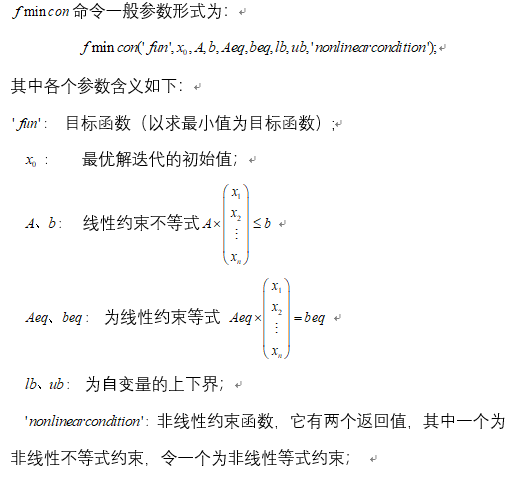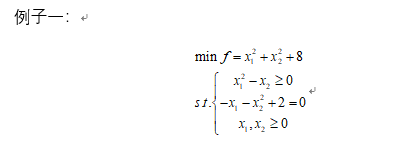%定义目标函数；
function f = myfun(x)
f = x(1)^2 + x(2)^2 + 8;
end

%非线性约束；
function [c, ceq] = nonlia(x)
c = -x(1)^2 + x(2);       %不等式约束；
ceq = -x(1) - x(2)^2 + 2; %等式约束；
end

%在命令窗口输入：
A = [];
b = [];
Aeq=[];
beq=[];
lb = [0; 0];
ub = [];
x0 = [0; 0];
[x,fval,exitflag,output,lambda,grad,hessian] = fmincon('myfun',x0,A,b,Aeq,beq,lb,ub,'nonlia');

得：x =

1.0000
1.0000

fval =

10.0000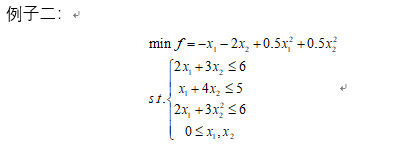%目标函数；
function f = myfun(x)
f = -x(1) - 2*x(2) +0.5*x(1)^2 + 0.5*x(2)^2;
end

%非线性约束；
function [c, ceq] = nonlia(x)
c = 2*x(1) + 3*x(2)^2 - 6;       %不等式约束；
ceq = []; %等式约束；
end

%在命令窗口输入：
clc;
clear all;
A = [2 3; 1 4];  %线性不等式系数；
b = [6;5];
Aeq = [];
beq = [];
lb = [0; 0];
ub = [];
x0 = [0; 0];
[x,fval,exitflag,output,lambda,grad,hessian] = fmincon('myfun',x0,A,b,Aeq,beq,lb,ub,'nonlia');

得：x =

0.7647
1.0588

>> fval

fval =

-2.0294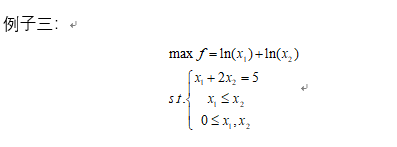注意这里是求最大值，目标函数需要取反；

%目标函数；
function f = myfun(x)
f = -(log(x(1)) + log(x(2)));
end

%非线性约束；
function [c, ceq] = nonlia(x)
c = [];       %不等式约束；
ceq = [];     %等式约束；
end

%在命令行输入：
clc;
clear all;
A = [1 -1];
b = 0;
Aeq= [1 2];
beq = 5;

lb = [0; 0];
ub = [];
x0 = [0; 0];
[x,fval,exitflag,output,lambda,grad,hessian] = fmincon('myfun',x0,A,b,Aeq,beq,lb,ub,'nonlia');

得：x =

1.6667
1.6667

>> fval

fval =

-1.0217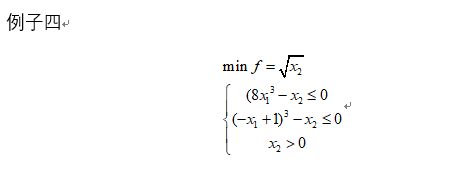%定义目标函数；
function f = myfun(x)
f = sqrt(x(2));
end

%非线性约束；
function [c, ceq] = nonlia(x)
c = [8*x(1)^3-x(2);(1-x(1))^3-x(2)];  %多个不等式约束情况；
ceq =[]; %等式约束；
end

%在窗口输入如下命令
A = [];
b = [];
Aeq = [];
beq = [];
lb = [0,0];
ub = [];
x0 = [1.234; 5.678];
[x,fval,exitflag,output,lambda,grad,hessian] = fmincon('myfun',x0,A,b,[],[],lb,[],'nonlia');

得：
x =

0.3333
0.2963

>> fval

fval =

0.5443

转载于:https://www.cnblogs.com/GuanghuiLiu/p/9931946.html
展开全文• 实验四 用 MATLAB 求解非线性优化问题 一实验目的 了解 Matlab 的优化工具箱利用 Matlab 求解非线性优化问题 二相关知识 非线性优化包括相当丰富的内容 们这里就 Matlab 提供的一些函数来介 绍相关函数的用法及其所...
• %线性规划 % [x,f]=linprog(c, A, b, Aeq, beq, lb, ub, options); %实数整数混合规划 % [x,f]=intlinprog(c, intcon, A,b,Aeq,beq,l,u); %二次规划 %opts = optimoptions('quadprog','Algorithm','...%非线性规划 % .
%线性规划
% [x,f]=linprog(c, A, b, Aeq, beq, lb, ub, options);
%实数整数混合规划
% [x,f]=intlinprog(c, intcon, A,b,Aeq,beq,l,u);
%二次规划
%opts = optimoptions('quadprog','Algorithm','interior-point-convex');
%[x,f] = quadprog(H,f,A,b,Aeq,beq,lb,ub,x0,options);
%非线性规划
% options = optimset('Algorithm', 'interior-point', 'Display', 'off');
%x=fmincon(fun, 初值, A,b,Aeq,beq,lb,ub,nonlcon, options)

%  [x,f]=fmincon(@fun, [0,0,1], [],[],[1,-1,1],,[],[],@nonlincon, options);
%
%  function fx=fun(x)
%  fx=x(1)^2+x(2)^4+x(3)^2-1;
%
%
%  end
% function [c, ceq] = nonlincon(x)
% c=x(1)^2+x(2)^2+x(3)^2-1;
% ceq = [ ];
% end


非线性优化fmincon最后是个例子上面是模板快展开全文• MATLAB求解非线性优化问题 实验四 用 MATLAB 求解非线性优化问题 一实验目的 了解 Matlab 的优化工具箱利用 Matlab 求解非线性优化问题 二相关知识 非线性优化包括相当丰富的内容我们这里就 Matlab 提供的一些...
• 使用优化实时编辑器任务的约束非线性规划示例。具有非线性不等式约束的非线性规划示例。Example of nonlinear programming with derivative information.使用所有导数信息的非线性规划示例。此示例说明如何求解具有...
基于有约束求解器的应用教程示例说明如何求解非线性问题和传递额外参数。使用优化实时编辑器任务的约束非线性规划示例。具有非线性不等式约束的非线性规划示例。Example of nonlinear programming with derivative information.使用所有导数信息的非线性规划示例。此示例说明如何求解具有线性或二次目标和二次不等式约束的优化问题。同时具有两类非线性约束的非线性规划。Example showing all constraints.Find the best feasible point in the outputstructure.Example showing efficiency gains possible with structured nonlinearproblems.Example showing nonlinear programming with only linear equalityconstraints.Example showing how to save memory in nonlinear programmingwith a structured Hessian and only linear equality constraints oronly bounds.Example showing how to calculate derivatives symbolically for optimizationsolvers.Use Symbolic Math Toolbox™ to generate gradients and Hessians.
展开全文• poblano_toolbox：MATLAB非线性优化
• 控制系统仿真课程设计 题 目基于 学生姓名 学 号 专 业 班 级 指导教师 NCD优化的非线性优化PID控制 内蒙古科技大学控制系统仿真课程设计 目录 基于NCD优化的非线性优化PID控制 . 4 摘 要 . 4 第一章 绪论 . 6 1.1 ...
• matlab程序非线性优化设计方法时下流行的关于非线性规划的源程序
• ## matlab_非线性优化

千次阅读 2015-08-19 11:44:59
matlab求解非线性问题 fmincon工具箱
• 非线性优化算法：各种非线性编程算法的MATLAB实现
• Matlab遗传算法工具箱在非线性优化中的应用-Matlab遗传算法工具箱在非线性优化中的应用.pdf 摘　要:投影寻踪是一种降维处理技术,通过它可以将多维分析问题通过投影方向转化为一维问题分析。应用该法的关 键在于...
• 非线性优化包括相当丰富的内容，我们这里就Matlab提供的一些函数来介绍相关函数的用法及其所能解决的问题。
• 多元函数的无约束最小值问题在Matlab中有2个经常使用的函数 1fminsearch 2fminunc;使用格式 [x,fval]=fminsearch@f,x0) 输入参数@f: 目标函数x0: 初始点(向量. 输出参数x: 最优解fval: 最优解对应的函数值. 例...
• snopt-matlab：用于稀疏非线性优化器SNOPT的Matlab接口
• matlab神经网络逼近函数不确定性，simulink中实现，可用于实现非线性控制中的未知函数逼近问题。
• 最近在做方程组的非线性优化问题，用到了fgoalattain函数，总结一下： 意义 解决多目标的非线性优化问题 函数形式 函数表示形式如下： 上式中，weight, goal, b和beq 是向量(组)，A 和Aeq 是矩阵, c(x), ceq(x)和F...
• 注意是最小化，其次约束是小于等于 编写约束函数先返回c再ceq 线性约束也写在非线性约束里会简化很多代码 例子 用的实时脚本 f = @(x)x(1)^2-2*x(1)+x(2); [a,b,c,d] = fmincon(f,[0;0],[],[],[],[],[],[],@ff) ...
• matlab机器学习 无约束非线性优化工具包 亲测有效 强大实用 提高效率缩短开发周期 非常好用 强烈推荐
• matlab开发-优化非线性控制估计工具箱。apm-matlab工具箱用于非线性控制和带ipopt的dae模型估计Simulink基础
• matlab开发-使用gmarguardt方法进行多变量非线性优化。基于马尔夸特方法的多元非线性优化
• 另外就是使用采用全局优化算法的软件(在理论上是不依赖于初值的)。不过楼主的问题似乎有过拟合现象导致目标函数值一样但参数组值不唯一。下面是用1stOpt计算得到的几组结果：1：均方差(RMSE): 0.00258526969626227......

# matlab非线性优化matlab 订阅